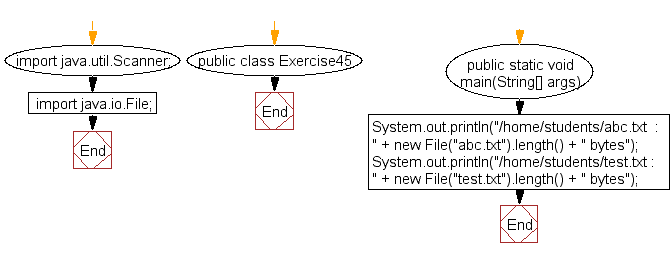﻿ Java exercises: Find the size of a specified file - w3resource# Java Exercises: Find the size of a specified file

## Java Basic: Exercise-45 with Solution

Write a Java program to find the size of a specified file.

Pictorial Presentation:Sample Solution:

Java Code:

``````import java.util.Scanner;
import java.io.File;
public class Exercise45 {

public static void main(String[] args) {
System.out.println("/home/students/abc.txt  : " + new File("abc.txt").length() + " bytes");
System.out.println("/home/students/test.txt : " + new File("test.txt").length() + " bytes");
}
}
```
```

Sample Output:

```/home/students/abc.txt  : 0 bytes
/home/students/test.txt : 0 bytes
```

Flowchart:Java Code Editor:

What is the difficulty level of this exercise?

Test your Programming skills with w3resource's quiz.

﻿

## Java: Tips of the Day

countOccurrences

Counts the occurrences of a value in an array.

Use Arrays.stream().filter().count() to count total number of values that equals the specified value.

```public static long countOccurrences(int[] numbers, int value) {
return Arrays.stream(numbers)
.filter(number -> number == value)
.count();
}
```

Ref: https://bit.ly/3kCAgLb